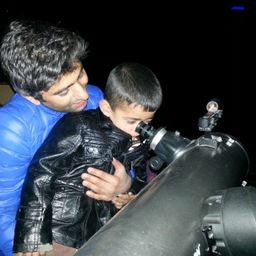Gravitation

### Discussion

You must be signed in to discuss.
##### Top Physics 101 Mechanics Educators##### Andy C.

University of Michigan - Ann Arbor##### Marshall S.

University of Washington##### Jared E.

University of Winnipeg

Lectures

Join Bootcamp

### Video Transcript

But let's say we have two ships A and be according to question, according to the given question Ah, proper lentil ship, eh? Uh, let's see, the proper lend off ship, eh is equal to three times off. Proper lentils should be, and we know old age L A is equal to L p times our square root off one minus weeks. Creator divided by C square. So contacted land l is the contract id land, and it's equal to properly and times square root off one minus B squared, divided by C square. So contacted land for ship, eh? Is written is l. A. Is equal to, uh, well, proper lent off ship, eh? Times Square, Rudolph One minus re squared, divided by C square. And similarly, the contract ID land for should be is equal to proper lend or should be Times Square. Load off one minus. Uh, we b squared, divided by C square. Here we Yeah. So this is the We is the velocity of ship air. And Bebe is the We lost your ship. Be. Let's say this is a question number one and this is acquitted. Number two. Uh, since Mont Lins are equal length off ship, eh? Contract id lent off ship, eh? Is it or Lent Measured by the Earth Observer for board ships. Oh, is equal. Therefore, L A is equal to l B. And we have Ah, proper lent off ship, eh? Proper length of huge ship. Eight times a square. Root off one minus. We a square divided by C square is equal to a proper lent. You should be Times Square, Rudolph One minus. We be square divided by C square. And we know of it. Um, proper length off ship is equal to three times a proper lentils should be, And therefore we have, ah, three times proper Lend off. Should be Times Square, Rudolph one minus we A square divided by C square is equal to proper lento. Should be times scared, Rudolph One minus. We be squared, divided by C square and simplifying uh, the airflow, um, mine into one minus. We A square divided by C square is equal to one minus. We be squared, divided by C Square, C square. And further we have we, uh we be, uh We be is Cuban. We lost. You should be is equal to, um zero point 350 times the speed of light cedar 0.3 50 times the speed of light. Let's substitute we be so we have nine into one minus a wee a square divided by C square is equal to one minus, uh, zero point pre 50 times. See whole screen divided by C square and solving for we eh? We have we A square divided by C square is equal to zero point zero point line Zito 25 on Therefore we eh divided by sea taking root on both sides You're 0.95 I need for we A is equal to zero point 95 times the speed of light. Therefore by ship is foster and it's the lost e Its speed is 0.95 times the speed of light.#### Topics

Gravitation

##### Top Physics 101 Mechanics Educators##### Andy C.

University of Michigan - Ann Arbor##### Marshall S.

University of Washington##### Jared E.

University of Winnipeg

Lectures

Join Bootcamp